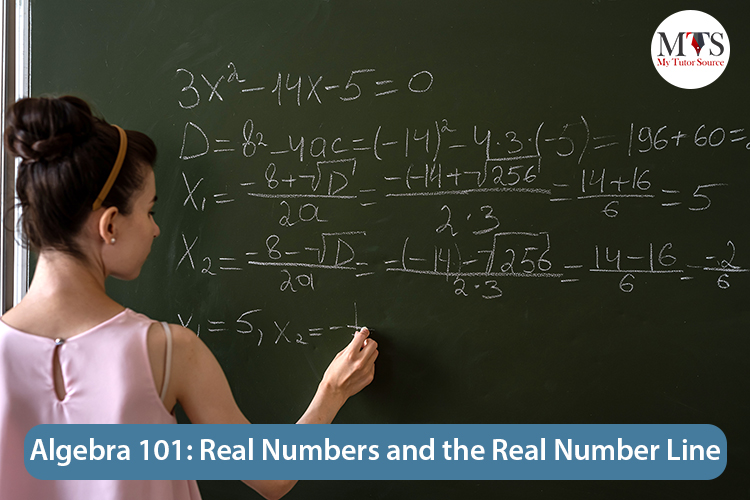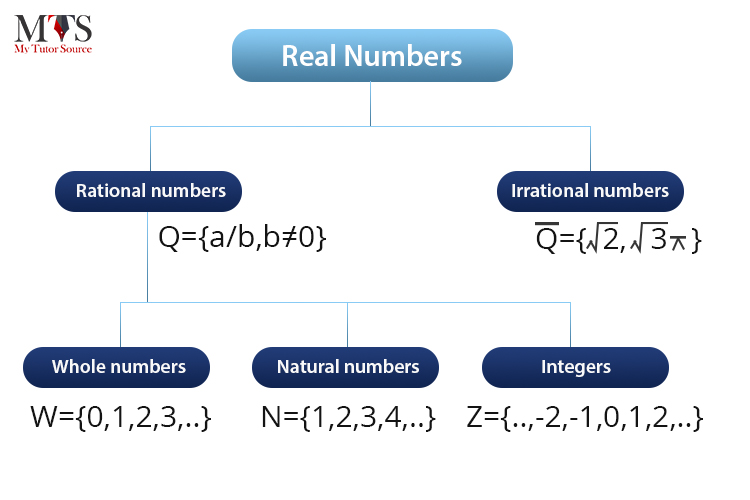# Algebra 101: Real Numbers and the Real Number Line## What Are Real Numbers?

Let’s start from the basics:

A set can be defined as a collection of objects that are grouped within parenthesis { }, and each object is known as an element. A subset is a set that has elements that are present in a given set. An empty set has no elements and it’s denoted as { } or ∅.
Sets are one of the main focuses in maths. The set of natural numbers is represented with N:
{1,2,3,4,5,…}
The three periods (…) is an ellipsis that shows that the numbers are infinite.
The set of whole numbers is represented with W. It consists of natural numbers and zero.
{0,1,2,3,4,5,…}
The set of integers has zero, positive and negative numbers. It’s represented as Z.
{…,−3,−2,−1,0,1,2,3,…}

The sets of whole and natural numbers are subsets of the integers’ set.Rational numbers are numbers in the form of ab, where b is non zero, and a and b are integers. They’re denoted as Q and b is nonzero. Decimals that terminate or repeat are rational.
0.7 = 710 AND 0.3 = 0.3333⋯ = 13

Irrational numbers are numbers that can’t be written in the form of a ratio of two integers. Non-repeating, non-terminating decimals are irrational.
π=3.14159… AND2 = 1.41421…
The set of real numbers is a set containing all rational numbers and all irrational numbers. It’s denoted as R. So, all the sets discussed above are subsets of the set of real numbers.
You can extend real numbers by adding the imaginary number i, which is equal to sqrt(-1) (-1). Moreover, numbers in the form of x+iy, where x and y are both real, also form a field, and are called complex numbers. Another extension is the surreal numbers that include both the infinite ordinal numbers of Georg Cantor and real numbers.

## Real Numbers Examples

• Natural numbers {1, 2, 3,…}
• Whole numbers {0, 1, 2, 3,…}
• Integers
• Decimal numbers
• Rational numbers
• Irrational numbers
• Integers Z {…, -2, -1, 0, 1, 2,…}
• 3/5
• 10.3
• 0.6
• 12/5
• 3.46466466646666…
• √ 2
• √ 3 are a few examples of real numbers.

## Properties of Real Numbers Definition

Let’s define the properties of real numbers:

## Closure Property

The product and sum of two real numbers is always another real number.

For all a, b ∈ R, a + b ∈ R, and ab ∈ R

## Associative Property

The product or sum or any three real numbers remains the same no matter how you change their grouping.

For all a,b,c ∈ R, a + (b + c) = (a + b) + c and a × (b × c) = (a × b) × c

## Commutative Property

The product or sum of two real numbers remains the same no matter how you interchange their order.

For all a, b ∈ R, a + b = b + a and a × b = b × a

## Distributive Property

The distributive property of multiplication over subtraction is a × (b – c) = (a × b) – (a × c) while the distributive property of multiplication over addition is is a × (b + c) = (a × b) + (a × c).

## Number Line

A real number line lets you display real numbers on a line by linking them to unique points. The real number linked to a point is known as a coordinate. While the point on the line that is linked to a coordinate is known as its graph.
To draw a real number line, you need to draw a horizontal line that has arrows on its both ends that indicate that the line continues without bound. Then choose a point to represent the zero. That point will be called the origin.
Mark consistent intervals on both sides of the number zero (origin) and label every tick mark (which represents one unit) to define your scale. Positive real numbers are always written on the right of the origin and the negative real numbers are written on the left. The origin is neither positive nor negative.
However, the scale doesn’t always have to be one unit. Every tick can represent two units or more. The graph of each real number is represented as a dot on the number line.

## Ordering Real Numbers

When you’ve to order real numbers on a number line, make sure that you place the bigger number to the right of the smaller number. For example, 20 is greater than 6, and -2 is greater than -7.

## Using Symbols

Symbols are used to effectively show the relationships between the numbers on the number line. For instance:

• = is equal to
• ≠ is not equal to
• ≈ is approximately equal to
These can be used in the following manner:

5 = 5

0 ≠ 5

π ≈ 3.145

Then come the symbols that represent an order relationship between real numbers, for example:

• < Less than
• > Greater than
• ≤ Less than or equal to
• ≥ Greater than or equal to
These can be used in the following manner:

−120 is placed at the left of –10 on the real number line, so it means that −120 is less than –10. You can write it as:

−120 < −10

The symbols < and > are used to show strict inequalities, while the symbols ≤ and ≥ are used to show inclusive inequalities. You can use more than one symbol in certain situations. Remember that you can only use the word ‘or’ when one of the given conditions is true: ‘equal to’ or ‘less than’, for example.

## An Example

Classify each given number as negative or positive and as either irrational or rational. Does the given number lie to the right or the left of the zero on the number line?

ⓐ −103

ⓑ 5

ⓒ −289

ⓓ −6π

ⓔ 0.615384615384…

Solution

ⓐ −103 is rational, negative, and lies on the left of the zero.

ⓑ 5 is irrational, positive, and lies on the right of the zero.

ⓒ −289= −17. It’s rational, negative, and lies on the left of the zero.

ⓓ −6π is irrational, negative, and lies on the left of the zero.

ⓔ 0.615384615384… is positive, rational, and lies on the right of the zero.

## Is 7 a Real Number?

Yes, 7 is a real number. The number 7 belongs to the set of natural numbers that also come under the real numbers.

## Is Zero a Real or an Imaginary Number?

Zero is both a real number and an imaginary number. Imaginary numbers are basically the square roots of non-positive real numbers. And because zero can also be considered a non-positive number, hence, it completes the criteria used to determine an imaginary number. On the other hand, zero is also a rational number because it can be defined on a number line. Therefore, it’s a real number.

That’s all for your Algebra lesson about real numbers. Best of luck!

### Find Top Tutors in Your AreaWith over 3 years of experience in teaching, Chloe is very deeply connected with the topics that talk about the educational and general aspects of a student's life. Her writing has been very helpful for students to gain a better understanding of their academics and personal well-being. I’m also open to any suggestions that you might have! Please reach out to me at chloedaniel402 [at] gmail.com